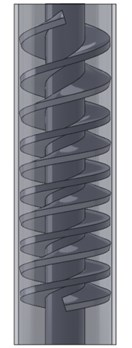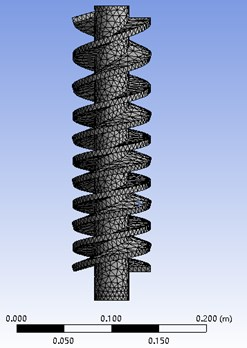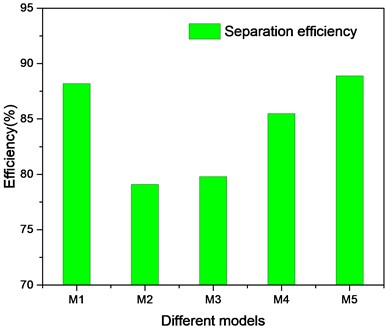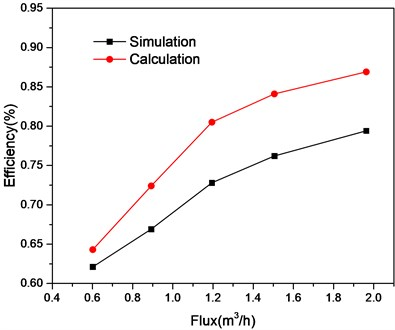# Study on separation characteristics of two-phase flow in double helical separator

## Hua Guo1, Chuangchuang Zhou2, Zhen Jiang3, Xiaojun Zhou4

1, 2, 3, 4School of Mechatronics Engineering and Automation, Shanghai University, Shanghai, China

1Shanghai Technical Institute of Electronics and Information, Shanghai, China

1Corresponding author

Vibroengineering PROCEDIA, Vol. 19, 2018, p. 274-278. https://doi.org/10.21595/vp.2018.20190
Received 1 September 2018; accepted 7 September 2018; published 24 September 2018

33rd International Conference on Vibroengineering in Zittau, Germany, September 24-26th, 2018

Copyright © 2018 Hua Guo, et al. This is an open access article distributed under the Creative Commons Attribution License, which permits unrestricted use, distribution, and reproduction in any medium, provided the original work is properly cited.
Views 252
CrossRef Citations 0
Abstract.

The helical separator plays an important role in improving the working efficiency of electric submersible pump. Separation efficiency of two-phase flow in double helical separator is studied by numerical simulation and theoretical calculation. It is found that the separation efficiency of helical separator increases with the increase of gas-liquid ratio and flow rate. At the same time, under the condition of constant helical number and gas-liquid ratio, the separation efficiency is best when the even difference of pitch is 10 mm.

Highlights
• The separation characteristics of gas-liquid two-phase flow in double helical separator are studied by numerical simulation and theoretical calculation.
• The effect of pitch on separation efficiency is analyzed and the separation efficiency is best when the even difference of pitch is 10mm with a certain helical numbers and gas-liquid ratio.
• When other conditions are constant, the separation efficiency increases with the increases of flow rate and gas-liquid ratio.

Keywords: separation efficiency, two-phase, helical separator, numerical simulation.

#### 1. Introduction

Helical separator is a kind of separation device which is widely used in the field of petroleum exploitation. The pitch of the conventional helical separator is a constant. Smaller pitch is conducive to separate gas and liquid. Larger pitch will increase flow resistance. The amount of fluid that wells produce is constantly changing and the output of each well is also different. If the design of separator and gas-liquid ratio are constant, which may not be suitable for another wells output. In addition, conventional helical separator has a single helical structure and the length of helical is shorter. To improve the oil and gas separation effect, the length of the helical must be increased [1, 2]. As the length of the helical increasing, the resistance of upward flow of the fluid increases. At the same time, the velocity of the liquid flow decreases, affecting the separation efficiency [3, 4]. Compared with single helical structure with the same helical pitch and the same helical length, double helical separator can improve the swirl velocity and effectively improve gas-liquid separation efficiency. In this study, the double helical separator is taken as the research object to study the separation efficiency in the separator.

According to the theory and method of computational fluid dynamics, using the FLUENT software to simulate the fluid flow in a helical separator and establishing the control equation of two-phase flow based on the flow field characteristics of separator. Suitable calculation model and meshing are chosen to investigate the two phase flow characteristics in double helical separator with variable pitch.

#### 2.1. Calculation model

Considering the characteristics of underground environment, the calculated model is simplified and the geometric model of double helical separator with variable pitch is established, as shown in Fig. 1. The outer diameter of helical separator pipe is 100 mm, the even difference of pitch is 10 mm and 15 mm, respectively. Total length is 530 mm and blade thickness is 10 mm-20 mm. For the convenience of calculation and the saving of calculation time and space, the computational fluid must be assumed and simplified. Assuming that the gas-liquid two-phase fluid is incompressible, and the flow is steady turbulent flow. When the fluid is static, the total pressure is static pressure. If the flow entering the separator comes from infinite distance, the inlet velocity is uniformly distributed. Ignoring the friction of pipe wall, the gas-liquid two-phase flow has no heat exchange with the outside environment and its temperature remains unchanged. The mesh generation of separator has great influence on the accuracy of calculation results. The principle of generating mesh is that the regions which are important to the study should be encrypted. In other areas, the distance between nodes can be enlarged appropriately, so that the calculation efficiency can be improved under the premise of ensuring the reliability of calculation. Fig. 2 shows the model grid partitioning method used in numerical calculation in this study. The working medium is water and air at normal temperature. The density of water is 1000 kg/m3 and the density of air is 1.37 kg/m3.

Fig. 1. Geometry of double helical separatorFig. 2. Mesh division of geometry model for double helical separator#### 2.2. Calculation model and boundary condition

In this study, the fluid in the helical separator is regarded as incompressible fluid. RNG $k$-$\epsilon$ model is a turbulence model established by Yakhot and Orzag  to correct the standard $k$-$\epsilon$ model. An additional item is added to the $\epsilon$ equation of the RNG $k$-$\epsilon$ model, increasing the accuracy of the fast flow calculation. In the RNG $k$-$\epsilon$ model, we consider the effect of vortex on turbulence, which is the opposite sex of turbulence, increasing the ability to predict rotational flow. At the same time, the coefficient in RNG $k$-$\epsilon$ model is calculated by theoretical formula rather than experience, so it is more adaptable. The equations of the turbulent kinetic energy and the dissipation rate of the turbulent kinetic energy are shown respectively:

(1)
$\frac{\partial \left(\rho k\right)}{\partial t}+\frac{\partial \left(\rho k{u}_{i}\right)}{\partial {x}_{i}}=\frac{\partial }{\partial {x}_{j}}\left({\alpha }_{k}{\mu }_{eff}\frac{\partial k}{\partial {x}_{j}}\right)+{G}_{k}+\rho \epsilon ,$
(2)
$\frac{\partial \left(\rho \epsilon \right)}{\partial t}+\frac{\partial \left(\rho \epsilon {u}_{i}\right)}{\partial {x}_{i}}=\frac{\partial }{\partial {x}_{j}}\left({\alpha }_{\epsilon }{\mu }_{eff}\frac{\partial \epsilon }{\partial {x}_{j}}\right)+\frac{{G}_{1\epsilon }^{\mathrm{*}}\epsilon }{k}{G}_{k}-{C}_{2\epsilon }\rho \frac{{\epsilon }^{2}}{k},$

where ${G}_{k}$ is the generating term of turbulent kinetic energy, model constant ${C}_{\mu }=$ 0.0845, ${\alpha }_{k}={\alpha }_{\epsilon }=$ 1.39, ${C}_{1\epsilon }=$ 1.42, ${C}_{2\epsilon }=$ 1.68, ${\eta }_{0}=$ 4.377, $\beta =$ 0.012.

The correlation between turbulent kinetic energy $k$ and turbulent kinetic energy dissipation rate $\epsilon$is still as follows:

(3)
${\mu }_{t}=\rho {C}_{\mu }\frac{{k}^{2}}{\epsilon }.$

It can be seen that the RNG $k$-$\epsilon$ activated model can be used for low Reynolds number flow. In the dissipation rate equation of turbulent kinetic energy, the time average strain rate term reflecting the mainstream is added, reflecting the influence of average strain rate on the dissipation term. Therefore, the RNG $k$-$\epsilon$ model can better handle the flow with high strain rate and large bend of streamline. In view of that the fluid in the helical separator is accompanied by the interaction between turbulence and helical flow, resulting in flow curve and local reflux, the RNG $k$-$\epsilon$ model is adopted in this study to simulate the fluid in the double helical separator.

#### 3.1. The influence of geometric parameters on separation efficiency

The helical number and pitch of the double helical gas-liquid separator with variable pitch are changed and the influence of structure parameters on the separation efficiency is studied by the method of simulation. Table 1 is double helical gas-liquid separator with variable pitch accompanied five different structure parameters. As shown in Fig. 3, the double helical separator with a flow rate of 1.982 m3/h has the highest separation efficiency when the helical number and gas-liquid ratio are constant. When the flow rate and the gas-liquid ratio are constant, the separation performance of the double helical separator with 10 mm even difference pitch is the best. The greater the gas-liquid ratio and the better the separation efficiency, when flow rate and pitch are constant.

Table 1. The structural parameters of the double helical gas-liquid separator

 Parameters M1 M2 M3 M4 M5 Helical numbers 5 6 7 7 7 Even pitch difference 10 15 15 15 15 Flow (m3/h) 1.125 1.125 1.982 1.982 1.982 Helical length (mm) 530 530 530 530 530 Leaf thickness (mm) 10 20 20 20 20 Gas-liquid ratio (%) 80 80 80 85 90

Fig. 3. Separation efficiency of different double helical structures#### 3.2. Separation efficiency at different flow rates

The model based on the mass and momentum balance equations was developed by Alhanati (1993) , which was used to predict the natural separation efficiency of two-phase flow. The expression of separation efficiency was expressed as:

(4)
$E=\frac{{v}_{\mathrm{\infty }}{\left(1-\alpha \right)}^{n}}{{v}_{sl}+{v}_{\mathrm{\infty }}{\left(1-\alpha \right)}^{n}},$
(5)
${v}_{\mathrm{\infty }}=\sqrt{2}{\left(\frac{\sigma \left({\rho }_{l}-{\rho }_{g}\right)g}{{\rho }_{l}^{2}}\right)}^{0.25},$

where ${v}_{sl}$ is the superficial velocity of the liquid phase, $\sigma$ is the surface tension, $n$ refers to the flow regime.

The separation efficiency under different flow rates with the same liquid content rate (the liquid holdup is 80 %) is shown in Table 2. It can be seen from Table 2 that the separation efficiency of double helical separator with variable pitch increases with the increase of flow rate. At the same flow, the numerical simulation data and theoretical calculation results of the separation efficiency of helical separator are similar and the maximum relative error is about 9.5 %. Both theoretical calculation and numerical simulation show that the cyclone intensity of the flow field inside the separator will also increase with the flow rate increases and the inlet velocity increases, which is conducive to the separation of gas-liquid two phases, as shown in Fig. 4.

Table 2. Separation efficiency at different flow rates

 Flux (m3/h) Separation efficiency of theoretical calculation Separation efficiency of numerical simulation Relative error (%) 0.602 0.621 0.643 3.42 0.893 0.644 0.694 7.60 1.195 0.708 0.815 9.57 1.506 0.732 0.841 9.40 1.963 0.767 0.869 8.63

Fig. 4. Relationship between separation efficiency and fluxIt can be found that the centrifugal motion of heavy liquid in helical separator and the centripetal motion of light component gas are the fundamental reasons for gas-liquid separation. The strong shear action in the shear layer near the solid wall makes bubbles bound in the liquid drop escape. In the separator, the pressure drops as the potential energy and kinetic energy of the mixed media increase, causing some small bubbles to expand, thus obtaining separated centripetal force, which is an important auxiliary factor for gas-liquid separation.

#### 4. Conclusions

The separation characteristics of gas-liquid two-phase flow in double helical separator are studied by numerical simulation and theoretical calculation. The effect of pitch on separation efficiency is analyzed and the separation efficiency is best when the even difference of pitch is 10 mm with a certain helical numbers and gas-liquid ratio. When other conditions are constant, the separation efficiency increases with the increase of flow rate and gas-liquid ratio.

#### Acknowledgements

This work was supported by Rapid Development of Mold based on RE/TDP Technology (Grant No. Z18051) and by Natural Science Foundation of China (Grant No. 51574161).

1. Juengcharoensukying J., Poochinda K., Chalermsinsuwan B. Effects of cyclone vortex finder and inlet angle on solid separation using CFD simulation. Energy Procedia, Vol. 138, 2017, p. 1116-1121. [Publisher]
2. Hosseini S. S., Farhadi M., Sedighi K. Experimental investigation of a solar desalination system using twisted tape and wire coil inside of spiral heat exchanger. Desalination, Vol. 420, 2017, p. 34-44. [Publisher]
3. Xu W., Li Q., Wang J., Jin Y. Performance evaluation of a new cyclone separator – Part II simulation results. Separation and Purification Technology, Vol. 160, 2016, p. 112-116. [Publisher]
4. Xiong Z., Ji Z., Wu X. Development of a cyclone separator with high efficiency and low pressure drop in axial inlet cyclones. Powder Technology, Vol. 253, 2014, p. 644-649. [Publisher]
5. Yakhot V. V., Orszag S. A. Renormalization-group analysis of turbulence. Physical Review Letters, Vol. 57, Issue 14, 1986, p. 1722-1724. [Publisher]
6. Alhanati, F. J. S. Bottom Hole Gas Separation Efficiency in Electrical Submersible Pump Installations. Ph.D. Thesis, University of Tulsa, Tulsa, 1993. [Search CrossRef]